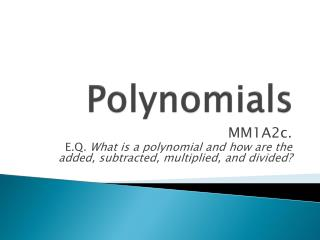DownloadDownload PresentationPolynomials

# Polynomials

Télécharger la présentation## Polynomials

- - - - - - - - - - - - - - - - - - - - - - - - - - - E N D - - - - - - - - - - - - - - - - - - - - - - - - - - -
##### Presentation Transcript

1. Polynomials MM1A2c. E.Q. What is a polynomial and how are the added, subtracted, multiplied, and divided?

2. Definitions • Polynomial – an expression formed by adding and subtracting terms of the form axn, where a is any number (coefficient) and n is a whole number. • Examples: • Monomial– an expression in the form of axn, where a is any number and n is a whole number, but there is only one term. • Examples:

3. Definitions continued • Binomial – an expression in the form of axn, where a is any number and n is a whole number, but there are two terms. • Examples: • Trinomial – an expression in the form of axn, where a is any number and n is a whole number, but there are threeterms. • Examples:

4. Complete the following 2x3 5x -11 4x7 -9x2 -15x 9x2 8 -22x3 2x7 Match up each monomial in the two columns and explain why you matched them together.

5. Adding/Subtracting Polynomials • Steps: 1. First identify like terms. 2. Look at only the coefficients to combine terms. 3. After all like terms are combined, put answer in standard form.